Updating search results...

# 9 Results

View
Selected filters:
• UT.MATH.I.G.CO.4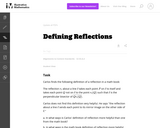Unrestricted Use
CC BY
Rating
0.0 stars

This is a task from the Illustrative Mathematics website that is one part of a complete illustration of the standard to which it is aligned. Each task has at least one solution and some commentary that addresses important asects of the task and its potential use. Here are the first few lines of the commentary for this task: Carlos finds the following definition of a reflection in a math book: The reflection $r_\ell$ about a line $\ell$ takes each point $P$ on $\ell$ to its...

Subject:
Mathematics
Secondary Mathematics
Material Type:
Activity/Lab
Provider:
Illustrative Mathematics
Provider Set:
Illustrative Mathematics
Author:
Illustrative Mathematics
07/09/2013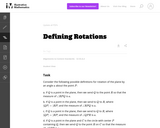Unrestricted Use
CC BY
Rating
0.0 stars

This is a task from the Illustrative Mathematics website that is one part of a complete illustration of the standard to which it is aligned. Each task has at least one solution and some commentary that addresses important asects of the task and its potential use. Here are the first few lines of the commentary for this task: Consider the following possible definitions for rotation of the plane by an angle $a$ about the point $P$: If $Q$ is a point in the plane, then we send...

Subject:
Mathematics
Secondary Mathematics
Material Type:
Activity/Lab
Provider:
Illustrative Mathematics
Provider Set:
Illustrative Mathematics
Author:
Illustrative Mathematics
07/09/2013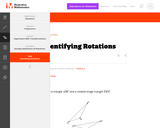Unrestricted Use
CC BY
Rating
0.0 stars

This is a task from the Illustrative Mathematics website that is one part of a complete illustration of the standard to which it is aligned. Each task has at least one solution and some commentary that addresses important aspects of the task and its potential use.

Subject:
Mathematics
Material Type:
Activity/Lab
Provider:
Illustrative Mathematics
Provider Set:
Illustrative Mathematics
Author:
Illustrative Mathematics
07/02/2018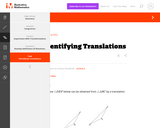Unrestricted Use
CC BY
Rating
0.0 stars

This is a task from the Illustrative Mathematics website that is one part of a complete illustration of the standard to which it is aligned. Each task has at least one solution and some commentary that addresses important aspects of the task and its potential use.

Subject:
Mathematics
Material Type:
Activity/Lab
Provider:
Illustrative Mathematics
Provider Set:
Illustrative Mathematics
Author:
Illustrative Mathematics
07/02/2018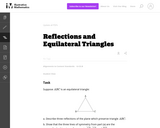Unrestricted Use
CC BY
Rating
0.0 stars

This activity is one in a series of tasks using rigid transformations of the plane to explore symmetries of classes of triangles, with this task in particular focusing on the class of equilaterial triangles. In particular, the task has students link their intuitive notions of symmetries of a triangle with statements proving that the said triangle is unmoved by applying certain rigid transformations.

Subject:
Mathematics
Secondary Mathematics
Material Type:
Activity/Lab
Provider:
Illustrative Mathematics
Provider Set:
Illustrative Mathematics
Author:
Illustrative Mathematics
01/05/2013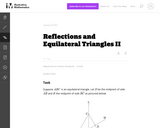Unrestricted Use
CC BY
Rating
0.0 stars

This task examines some of the properties of reflections of the plane which preserve an equilateral triangle: these were introduced in ''Reflections and Isosceles Triangles'' and ''Reflection and Equilateral Triangles I''. The task gives students a chance to see the impact of these reflections on an explicit object and to see that the reflections do not always commute.

Subject:
Mathematics
Secondary Mathematics
Material Type:
Activity/Lab
Provider:
Illustrative Mathematics
Provider Set:
Illustrative Mathematics
Author:
Illustrative Mathematics
01/05/2013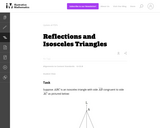Unrestricted Use
CC BY
Rating
0.0 stars

This activity is one in a series of tasks using rigid transformations of the plane to explore symmetries of classes of triangles, with this task in particular focussing on the class of isosceles triangles.

Subject:
Mathematics
Secondary Mathematics
Material Type:
Activity/Lab
Provider:
Illustrative Mathematics
Provider Set:
Illustrative Mathematics
Author:
Illustrative Mathematics
01/05/2013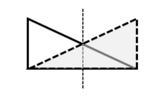Conditional Remix & Share Permitted
CC BY-NC
Rating
0.0 stars

This is a lesson plan that introduces rotational and reflectional symmetry with geometric shapes, focusing on regular polygons. Notes (two copies: a student version and also an answer key, both as a Word doc and a PDF), assignment, and cut-out shapes are provided.

Subject:
Secondary Mathematics
Material Type:
Homework/Assignment
Lesson
Author:
Kimberly
02/07/2022Conditional Remix & Share Permitted
CC BY-NC
Rating
0.0 stars

This is a lesson plan that introduces rotational and reflectional symmetry with geometric shapes, focusing on regular polygons. Notes (two copies: a student version and also an answer key, both as a Word doc and a PDF), assignment, and cut-out shapes are provided. There is a final project, with both instructions and an example included. Cover image: Christensen, K., 2022. Reflectional Symmetry of an Atom [screenshot] (Keynote presentation, Rotational and Reflectional Symmetry Assignment).

Subject:
Secondary Mathematics
Material Type:
Activity/Lab
Assessment
Diagram/Illustration
Homework/Assignment
Lesson Plan
Author:
Kimberly